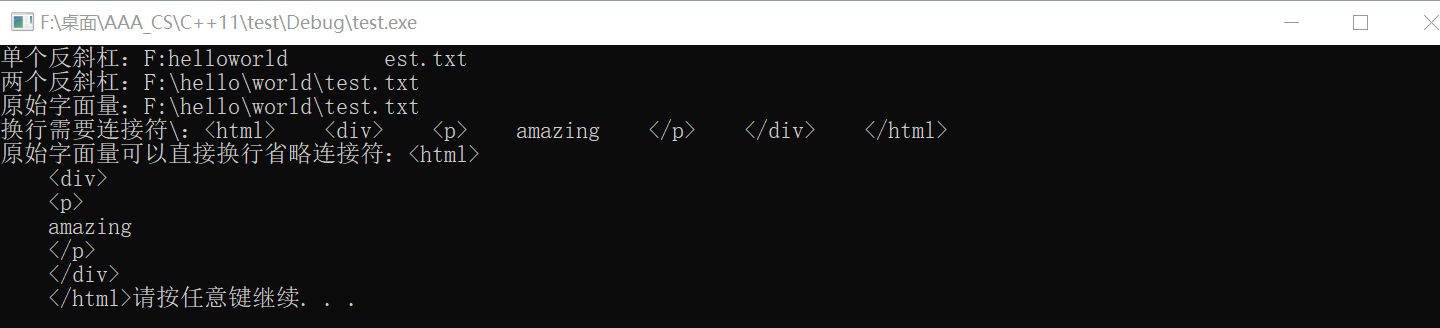# c++11_原始字面量

2022-05-14 22:09 1871 查看

# 1. 目的

• 解决字符串中被错认为是转义字符或其他特殊字符的问题
• 优雅地输出多行字符串

# 2. 格式

`R"xxx()xxx"`

`xxx`

# 3. 例子

```//原始字面量R"xxx()xxx"
#include <iostream>
#include<string>

using namespace std;
int main() {
string str = "F:\practice\test.txt";
cout << "一个反斜杠：" << str << endl;
string str1 = "F:\\practice\\test.txt";
cout << "两个反斜杠：" << str1 << endl;
string str2 = R"("F:\practice\test.txt")";
cout << "原始字面量：" << str2 << endl;

string str3 = R"(<html>
<div>
<p>
amazing
</p>
</div>
</html>)";
cout << "原始字面量多行字符串：" << str3;
system("pause");
return 0;
}```# 4. 注意事项

• 括号前后注释必须相同，可以省略，建议省略
• 如果必须写注释，注释中不要带括号，否则可能会出错。
• 括号内的内容里可以出现括号 总结：不要注释，括号内可以含有括号